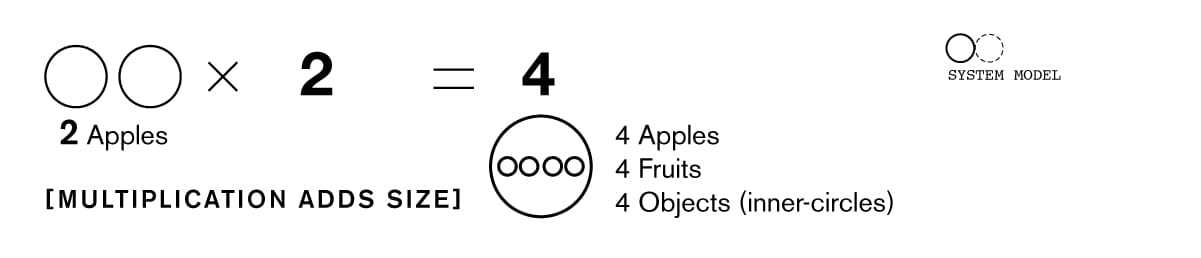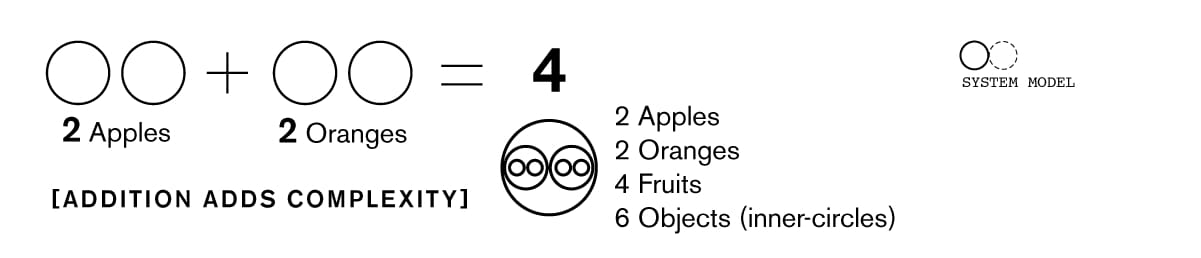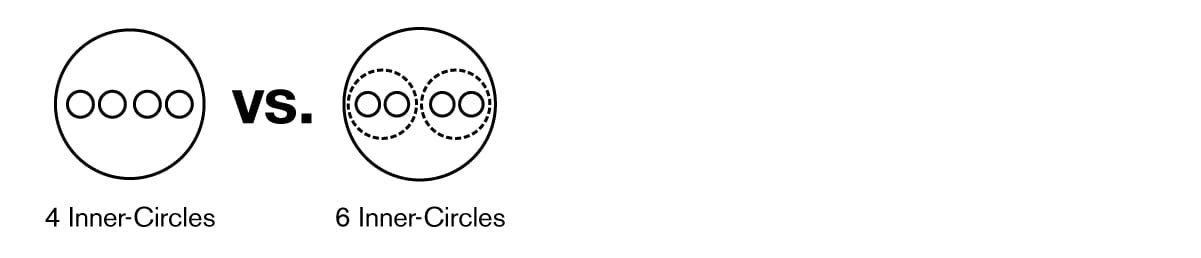# See the difference between 2x2 and 2+2

Let's examine the difference between 2x2 and 2+2, but from a different perspective!

Both equations equal 4, however there is a real difference between the two results. The fundamental difference springs from the operator used (addition '+' vs. multiplication 'x').

Here is the logic behind multiplication:In multiplication, objects are copied a number of times. Multiplication increases the size of a symbol, without increasing its complexity.

On the other hand, here is the logic behind addition:During addition, two different objects are combined into a single symbol. Each group of objects remains a distinct entity within the symbol, and thus increases its overall complexity.

Multiplication scales up a single group, while addition merges two different groups together and creates additional layers of 'depth'. Although both operations result in the same numerical result, the operations yield very different underlying shapes and meanings.

Ok, so what does this difference truly mean?...

When we calculate conventionally, we only see the 4 physical objects, while ignoring the other 2 abstract containers.From an abstract perspective, 2+2 = 6 (circles). The additional hidden circles are stores of real values within mindspace and can will be accessed by the mind when making logical decisions.

This hidden source of value, recognized as meaningful differences, is like an additional source of energy that motivates human beings.

Mindspace contains huge numbers of these hidden pockets, and symbols gain a large portion of their power from them.